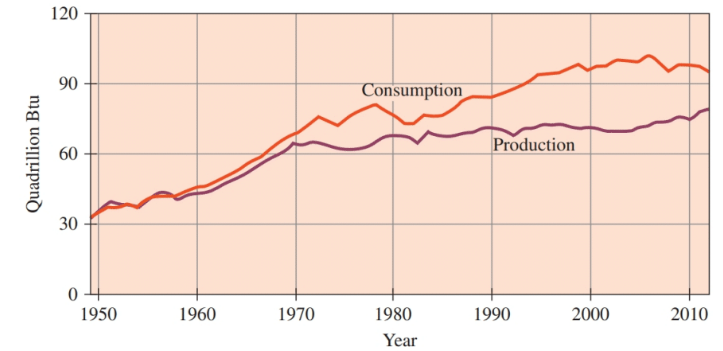# In the 1950s, the United States produced about the same amount of energy that it consumed. However, since then, a gap has grown between consumption and production. Today, the United States produces less energy than it consumes, and the difference is met by imported energy, mostly petroleum. Consider the following graph: a. Estimate the increase in energy consumption (in quads) between 1949 and 2012. What is the average yearly increase during this period? b. Estimate the increase in energy production (in quads) between 1949 and 2012. What is the average yearly increase during this period? c. What is the gap (in quads) between production and consumption in 2012?### Chemistry In Focus

7th Edition
Tro + 1 other
Publisher: Cengage Learning,
ISBN: 9781337399692

#### Solutions

Chapter
Section### Chemistry In Focus

7th Edition
Tro + 1 other
Publisher: Cengage Learning,
ISBN: 9781337399692
Chapter 9, Problem 57E
Textbook Problem
5 views

## In the 1950s, the United States produced about the same amount of energy that it consumed. However, since then, a gap has grown between consumption and production. Today, the United States produces less energy than it consumes, and the difference is met by imported energy, mostly petroleum. Consider the following graph:a. Estimate the increase in energy consumption (in quads) between 1949 and 2012. What is the average yearly increase during this period?b. Estimate the increase in energy production (in quads) between 1949 and 2012. What is the average yearly increase during this period?c. What is the gap (in quads) between production and consumption in 2012?

Interpretation Introduction

Interpretation:

Increase in energy consumption between 1949 and 2012 is to be estimated and the average yearly increase during this period is to determined. The increase in energy production between 1949 and 2012 is to be estimated and the average yearly increase during this period is to be determined. The gap between the production and the consumption in 2012 are to be calculated.

Concept Introduction:

Energy is the property that is required by an object to perform work and to heat itself and it can be measured.

The average change is calculated by dividing the change in the value by the elapsed time.

### Explanation of Solution

a) The increase in the energy consumption between 1949 and 2012 and the average yearly increase during this period.

The average energy consumption between 1949 and 2012 is calculated as follows:

Theyearlyaverageconsumption=Energyconsumptionin2012Energyconsumptionin194920121949

Substitute the value of energy consumption in 1949 and 2012 in the above expression.

Hence, the average yearly energy consumption between 1949 and 2012 is,

b) The increase in energy production between 1949 and 2012 and average yearly increase during this period.

The average energy production between 1949 and 2012 is calculated as follows:

### Still sussing out bartleby?

Check out a sample textbook solution.

See a sample solution

#### The Solution to Your Study Problems

Bartleby provides explanations to thousands of textbook problems written by our experts, many with advanced degrees!

Get Started

Find more solutions based on key concepts
For managing type 2 diabetes, regular physical activity can help by redistributing the body's fluids. T F

Nutrition: Concepts and Controversies - Standalone book (MindTap Course List)

Tell how you would try to prove to a doubter that air is matter.

Chemistry for Today: General, Organic, and Biochemistry

Why isnt tidal power being developed more aggressively?

Oceanography: An Invitation To Marine Science, Loose-leaf Versin

In an earthquake, both S (transverse) and P (longitudinal) waves propagate from the focus of the earthquake. Th...

Physics for Scientists and Engineers, Technology Update (No access codes included)

What is the argon core? What is its symbol, and what do you use it for?

Introductory Chemistry: An Active Learning Approach